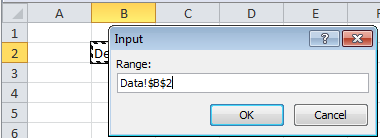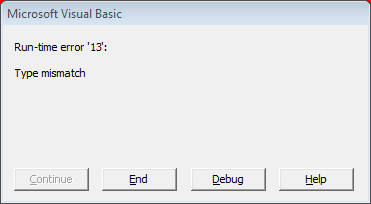﻿ Set range variable using VBA in Excel# Set range variable using VBA in Excel

Setting a range variable is done by using a procedure that returns a range. Ranges being the core of Excel, VBA provides many such procedures. The major ones are discussed here.

Note
Normally, setting a range does not change the active range. This only happens with the Select and Activate methods - whose use is generally considered harmful / should be avoided..

## Using the Range methods

### Application.Range - general use

The code below returns [Book2]Sheet2!\$A\$3:\$B\$5 in the Immediate window. It shows you can specify any range in an open workbook on any worksheet.

``````Dim rng As Range 'Declares a variable rng of type Range'
Set rng = Application.Range(Cell1:="[Book2]Sheet2!A3:B5")
'The Set keyword is required to assign an object to a variable (such as range)'
Debug.Print "[" & rng.Worksheet.Parent.Name & "]" & rng.Worksheet.Name & "!" & rng.Address``````

If in the above you leave out the worksheet or workbook the Application.Range method will assume the active one:

`Application.Range("Sheet2!A3:B5")` - in the active workbook
`Application.Range("A3:B5")` - in the active worksheet

The argument Cell1 requires the name of the range. It can be a Range object that contains a single cell, an entire column, or entire row, or it can be a string that names a single cell in the language of the macro.

Note
If the workbook or worksheet is not present running the code will give runtime Error 1004 “Application-defined or Object-defined error”

### Range - topleft and bottomright

Range has two arguments which are used to indicate the area in a worksheet. In the previous section only Cell1 was used. If the Optional Cell2 argument is also used Cell1 is the cell in the upper-left and Cell2 in the lower-right corner of the range.``````Dim rng As Range
Set rng = Application.Range(Cell1:="B2", Cell2:="D4")``````

### Select Range using the range selection dialog

When you need to specify the range, the easiest is to use the Code VBA range selection dialog. On opening this only shows the range part \$B\$2. You can include the sheetname Data! by explicitly switching to that sheet.### Worksheet.Range

In the previous section the the .Range property was called from the Application object. When writing business code it generally is better to be specific about which workbook and which sheet you are operating on. These will be normally be already be available as variables in your code. Below example uses the named range "Company" which makes it clearer what data is contained in the range.

``````Dim wb As Workbook: Set wb = ThisWorkbook
Dim ws As Worksheet: Set ws = wb.Sheets("Sheet1")
Set rng = ws.Range(Cell1:="Company")``````

### Worksheet.UsedRange

UsedRange returns a Range object representing the area of a worksheet - read more ...- that is being used in a broad sense including data, formatting and other uses.

### Set Range from another Range

Considering that a range object is the most specific indication of where to start looking, there are quite a few methods that use it to obtain a new range.

#### Range.Offset

Set a range with position relative a starting range. Positive values are offset downward, and negative values are offset upward. As an example, the code below prints \$D\$3. 2 rows down (RowOffset) and 3 columns to the right (ColumnOffset) of the start position A1.

``````Dim rngOffset As Range
Dim rng As Range: Set rng = Application.Range("\$A\$1")
Dim iRowOffset As Integer: iRowOffset = 2
Dim iColumnOffset As Integer: iColumnOffset = 3
Set rngOffset = rng.Offset(RowOffset:=iRowOffset, ColumnOffset:=iColumnOffset)

#### Range.Resize

Range.Resize resizes the specified range. `RowSize` specifies the number of rows in the new range, `ColumnSize` the number of columns. When either argument is omitted, the number of rows or columns in the range remains the same.

##### Use Resize to remove the top row

The code below removes the top row. This is useful when you need work with a table, without the header row.

``````Dim rng As Range: Set rng =
Dim rngTopRowRemoved As Range
Set rngTopRowRemoved = rng.Offset(1, 0).Resize(rng.Rows.Count - 1, rng.Columns.Count)``````

The resize which is one row smaller `Rows.Count - 1` would return the current range minus the bottom row. That's why in the above code, first the range is taken 1 row down `rng.Offset(1, 0)`.

### Selection in active worksheet - or window

In general purpose macros - such as copy to clipboard, which are supposed to work on any range, the common way to refer to the active range is Selection:

``Set rng = Application.Selection``

The returned object type depends on the current selection. For example, if a cell is selected, this property returns a Range object, if however a rectangle-Shape is selected an object of type Rectange is returned. If this is a possible scenario, two approaches are possible, discussed in the next sections.#### Handle error 13: Type mismatch

If a shape was selected instead of a range, using the above code with no error handling in place would result in the error dialog, and the user even maybe entering your code. To prevent this, you should add error handling.

#### Check if selection has correct object type

alternatively, you can use an object variable, and make sure that it is the correct type before continuing.

``````Dim obj As Object
Set obj = Application.Selection
If TypeName(obj) <> "Range" Then
Exit Sub
End If
Dim rngSelection As Range
Set rngSelection = obj``````

### Intersection of two or more ranges

`Application.Intersect` returns a Range object that represents the intersection of two or more ranges. In the example below the address of rngIntersect is B2:C3. If the ranges have no intersection the `rngIntersect Is Nothing`

``````Dim rngArg1 As Range: Set rngArg1 = Application.Range("Sheet1!A1:C3")
Dim rngArg2 As Range: Set rngArg2 =rngArg1 = Application.Range("Sheet1!B2:D4")
Dim rngIntersect As Range
Set rngIntersect = Application.Intersect(Arg1:=rngArg1, Arg2:=rngArg2)``````
Note
If one or more ranges from a different worksheet are specified, run-time error '1004': Method 'Intersect' of object '_Application' failed will be returned.

### Union of two or more ranges.

`Application.Union returns the union of two or more ranges.` In the example below the address of rngUnion for A1:C3 and C3:D4 is A1:C4 and the count is 12, which suggests the range can be used as expected when iterating over cells - even though the third row was overlapping.

``````Dim rngArg1 As Range: Set rngArg1 = Application.Range("Sheet1!A1:C3")
Dim rngArg2 As Range: Set rngArg2 = Application.Range("Sheet1!A3:C4")
Dim rngUnion As Range
Set rngUnion = Application.Union(Arg1:=rngArg1, Arg2:=rngArg2)``````

If the area can't unite nicely into 1 rectangle, the address becomes compound. The union of A1:C3 and B2:D4 has the address A1:C3,B2:D4 and count is 18. This shows that the cells in the overlap are interpreted as occurring twice!